# Hashing Hyperplane Queries to Near Points with Applications to Large-Scale Active Learning

Prateek Jain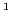, Sudheendra Vijayanarasimhan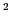, and Kristen GraumanMicrosoft Research Lab, Bangalore, INDIA,
University of Texas, Austin, TX, USA## Motivation

Goal: For large-scale active learning, want to repeatedly query annotators to label the most uncertain examples in a massive pool of unlabeled data.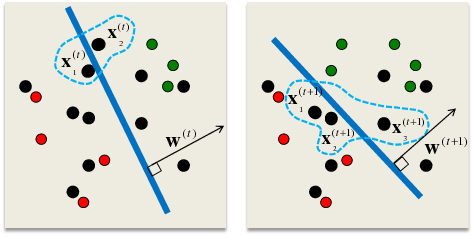Margin-based selection criterion for SVMs [Tong & Koller, 2000] selects points nearest to current decision boundary: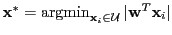Problem: With massive unlabeled pool, we cannot afford exhaustive linear scan.

## Main Idea: Sub-linear Time Active Selection

Idea: We define two hash function families that are locality-sensitive for the nearest neighbor to a hyperplane query search problem. The two variants offer trade-offs in error bounds versus computational cost.• Offline: Hash unlabeled data into table.
• Online: Hash current classifier as query" to directly retrieve next examples for labeling.

Main contributions:

• Novel hash functions to map query hyperplane to near points in sub-linear time.
• Bounds for locality-sensitivity of hash families for perpendicular vectors.
• Large-scale pool-based active learning results for documents and images, with up to one million unlabeled points.

## Background: Locality-Sensitive Hashing (LSH)

Let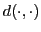be a distance function over items from a set, and for any item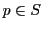, let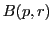denote the set of examples fromwithin radius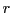from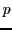.

Definition:

Letdenote a random choice of a hash function from the family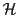. The familyis calledsensitive forwhen, for any,

• ifthen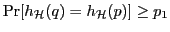,
• if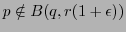then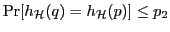.

Algorithm:

• Compute-bit hash keys for each point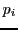: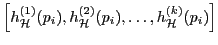.
• Given a query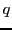, search over examples in the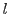buckets to whichhashes.
• Use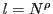hash tables for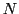points, where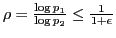,
• A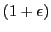-approximate solution is retrieved in time.

## First Solution: Hyperplane Hash

Intuition: To retrieve those points for which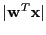is small, we want collisions to be probable for vectors perpendicular to hyperplane normal (assuming normalized data).For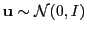,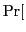signsign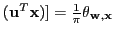[Goemans & Williamson, 1995].

Our idea: Generate two independent random vectors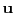and: one to capture angle between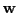and, and one to capture angle between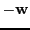and.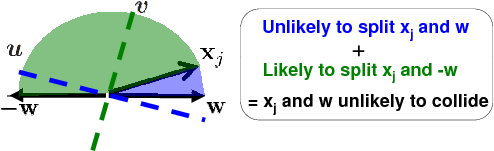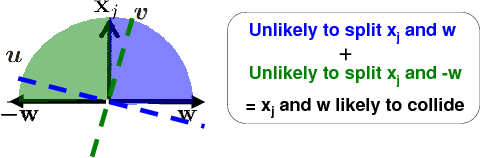Definition: We define H-Hash function familyas: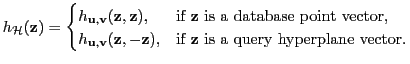where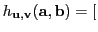sign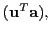sign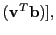is a two-bit hash, and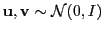.

Analysis:

• Probability of collision betweenandis given by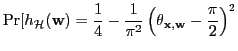• Hence, can return a point for which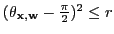in sub-linear time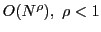.

## Second Solution: Embedded Hyperplane Hash

Intuition: Design Euclidean embedding after which minimizing distance is equivalent to minimizing, making existing approx. NN methods applicable.

Definition: We define EH-Hash function familyas: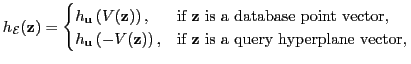where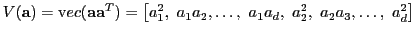gives the embedding, andsign, with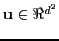sampled from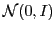.

Analysis:

• Since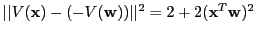, distance between embeddings ofandproportional to desired distance, so standard LSH functionapplicable.
• We have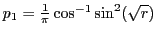. Hence, sub-linear time search with about twice the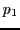guaranteed by H-Hash.

Issue:is-dimensional, higher hashing overhead.

Solution: Compute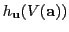approximately using randomized sampling:

H-Hash has faster pre-processing, but EH-Hash has stronger bounds.

 H-Hash: EH-Hash: Accuracy Hashing insertion time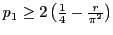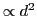(with sampling)

## Experimental Results

Goal: Show that proposed algorithms can select examples nearly as well as the exhaustive approach, but with substantially greater efficiency.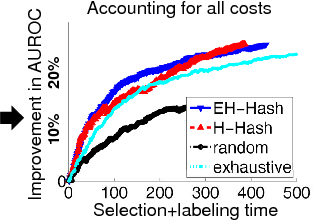Newsgroups: 20K documents, bag-of-words features.Tiny Images: 60K-1M images, Gist features.

• Accounting for both selection and labeling time, our approach performs better than either random selection or exhaustive active selection.
• Trade-offs confirmed in practice: H-Hash faster, EH-Hash more accurate.
• In future work, we plan to explore extensions for non-linear kernels.

## Publication

Hashing Hyperplane Queries to Near Points with Applications to Large-Scale Active Learning,
P. Jain, S. Vijayanarasimhan and K. Grauman, in NIPS 2010
[paper, supplementary]